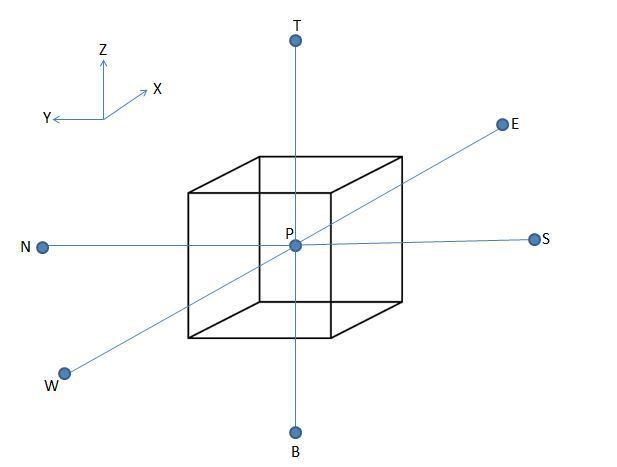# Finite volume method for three dimensional diffusion problem

Updated on
Covid-19Finite volume method (FVM) is a numerical method. FVM in computational fluid dynamics is used to solve the partial differential equation which arises from the physical conservation law by using discretisation. Convection is always followed by diffusion and hence where convection is considered we have to consider combine effect of convection and diffusion. But in places where fluid flow plays a non-considerable role we can neglect the convective effect of the flow. In this case we have to consider more simplistic case of only diffusion. The general equation for steady convection-diffusion can be easily derived from the general transport equation for property ϕ by deleting transient.

General transport equation is defined as:

ρ ϕ t + div ( ρ ϕ υ ) = div ( Γ grad ϕ ) + S ϕ …………………………………………….1

Where,

ϕ is a conservative form of all fluid flow,

ρ is density,

div ( ρ ϕ υ ) is a net rate of flow of ϕ out of fluid element represents convective term,

ρ ϕ t is a transient term,

div ( Γ grad ϕ ) is a rate of change ϕ of due to diffusion,

S ϕ is a rate of increase of ϕ due to source.

Due to steady state condition transient term becomes zero and due to absence of convection convective term becomes zero, therefore steady state three- dimensional convection and diffusion equation becomes:

div ( Γ grad ϕ ) + S ϕ = 0 ………………………………………………………….2

Therefore,

x ( Γ ϕ x ) + y ( Γ ϕ y ) + z ( Γ ϕ z ) + S ϕ = 0 …………………………………………………………………….3

Flow should also satisfy continuity equation therefore,

( ρ u ) = 0 ………………………………………………………………………………………………………4

## To solve the problem we will follow following general steps

Grid formation:

1. Divide the domain into discrete control volume.

2. Place the nodal point between end points defining the physical boundaries. Boundaries/ faces of the control volume are created midway between adjacent nodes.

3. Set up the control volume near the edge of domain such that physical as well as control volume boundaries will coincide with each other.

4. Considering a general nodal point P accompanied by six neighboring nodal point ‘E’ (which represent east), ‘W’ (which represent west), ‘N’ (which represent north), ‘S’ (which represent south), ‘T’ (which represent Top), ‘B’ (which represent bottom). In the considered control volume east side face is referred by ‘e’, west side face is referred by ‘w’, north side face is referred by ‘n’, south side face is referred by ‘s’, top side face is referred by ‘t’, bottom side face is referred by ‘b’.

5. Now the distance between nodes W and P, between nodes P and E, between nodes P and N, between nodes S and P, between nodes P and T, between nodes B and P are denoted as δ x W P , δ x P E , δ x P N , δ x S P , δ x P T , δ x B P respectively.

Discretisation:

On integration of equation 3 in one dimension over the general control volume gives:

[ ( Γ A d ϕ d x ) e ( Γ A d ϕ d x ) w ] + [ ( Γ A d ϕ d x ) n ( Γ A d ϕ d x ) s ] + [ ( Γ A d ϕ d x ) t ( Γ A d ϕ d x ) b ] + S Δ V = 0

Now using central differencing method we can rewrite above equation as

[ Γ e A e ( ϕ E ϕ P δ x P E ) Γ w A w ( ϕ P ϕ W δ x P E ) ] + [ Γ n A n ( ϕ E ϕ P δ x P E ) Γ s A s ( ϕ P ϕ W δ x P E ) ] + [ Γ t A t ( ϕ E ϕ P δ x P E ) Γ b A b ( ϕ P ϕ W δ x P E ) ] + ( S u + S p ϕ p ) = 0

This can be rearranged to give the discretised equation for interior nodes:

a P ϕ P = a W ϕ W + a E ϕ E + a S ϕ S + a N ϕ N + a B ϕ B + a T ϕ T + S u

Where

Solution of equation:

1. For solving the one- dimensional convection- diffusion problem we have to express equation (8) at all the grid nodes.

2. Now obtained set of algebraic equations is then solved to obtain the distribution of the transported property ϕ .

## References

Similar Topics
Jodidar
Soufian Benyamina
Babette March
Topics

 B i Link H2
 L